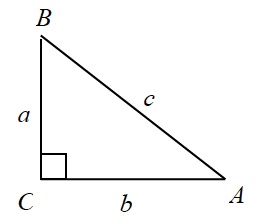### Home > A2C > Chapter 13 > Lesson 13.1.4 > Problem13-70

13-70.

Using the triangle on the right, find:1. $\sin A$

Remember that $\sin(x)$ is opposite over hypotenuse.

$\sin \left(A\right)\ =\ \frac{a}{c}$

1. $\sec A$

How is $\sec(x)$ related to $\cos(x)?$

1. $\csc B$

Which trigonometric ratio is $\csc(x)$ related to? How is it related?

1. $\tan B$

Remember that $\tan(x)$ is opposite over adjacent.